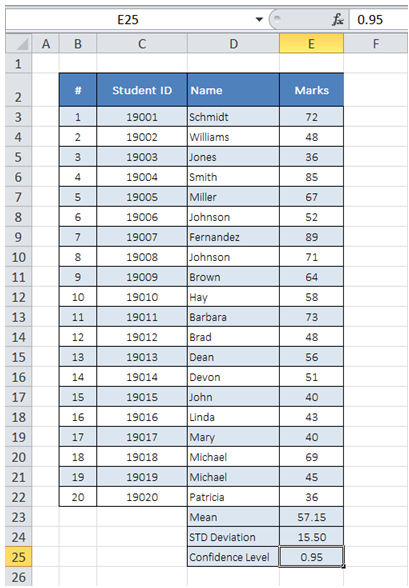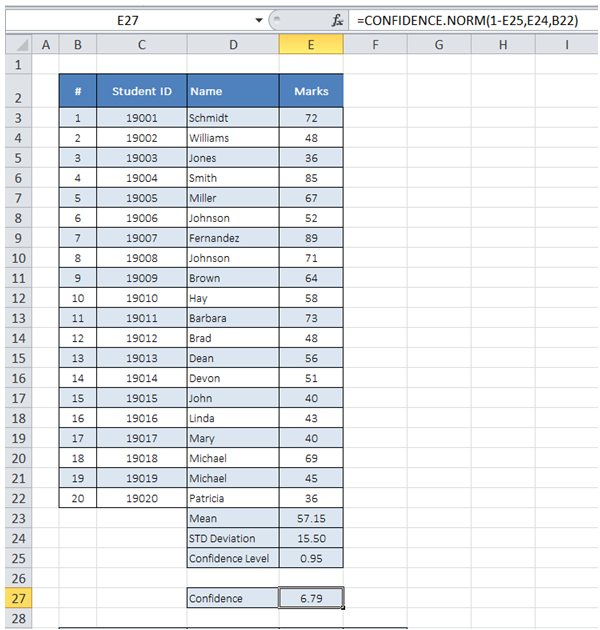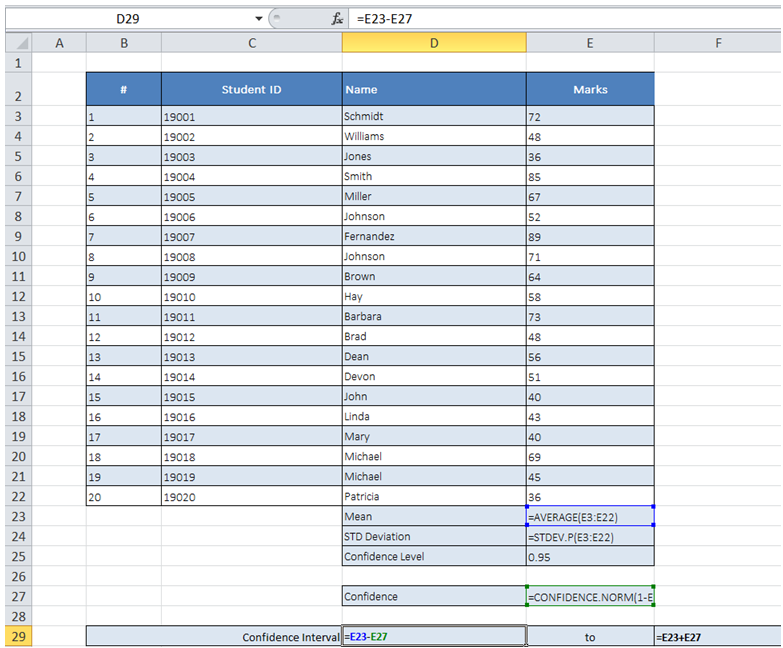Confidence interval of population in Excel

As shown in Table below, I have test results for my entire class captured. I have calculated Population Mean and Standard Deviation. I want to determine confidence interval for the calculated

Population Mean with a 95% confidence level.To do it in Excel, here is the answer:

a) Enter the formula =CONFIDENCE.NORM(1-E25,E24,B22) as shown below to get the confidence value

The first argument is a which is "1-Confidence Level". Second argument is Standard Deviation and third argument is population size.

The ".NORM" in formula indicates interval is calculated using a Normal distribution.b) Subtract and add confidence value to mean to get the lower and upper limit of Confidence Interval as shown below.